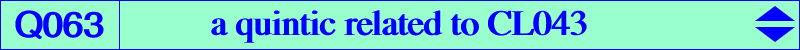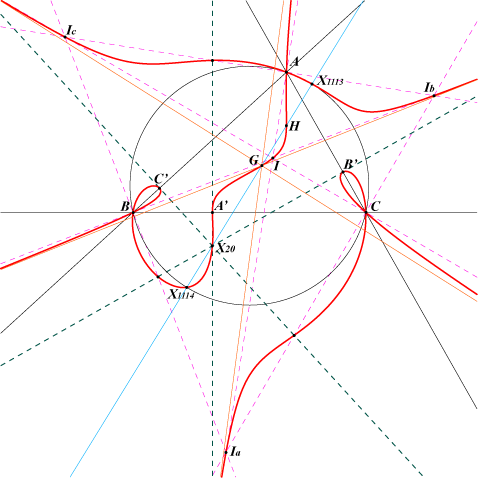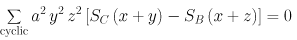X(1), X(2), X(4), X(20), X(1113), X(1114) excenters infinite points of the Thomson cubic circular points at infinity vertices of the pedal triangle of X(20) foci of the inconic with center O, perspector X(69)Q063 is the locus of pivots P of isogonal pKs meeting the circumcircle at A, B, C and three other points where the tangents are concurrent. See CL043. For example, with P = X(2), X(4), X(20), we obtain the Thomson cubic, the Orthocubic, the Darboux cubic. Q063 is a circular quintic with singular focus X(376), the reflection of G in O. Q063 has three real asymptotes parallel to those of the Thomson cubic and concurrent at G. A, B, C are nodes and the fifth points on the sidelines of ABC are the vertices A', B', C' of the pedal triangle of X(20). The tangents at these points pass through X(20) and meet the corresponding bisectors at six points on the curve. The isogonal transform of Q063 is Q113.